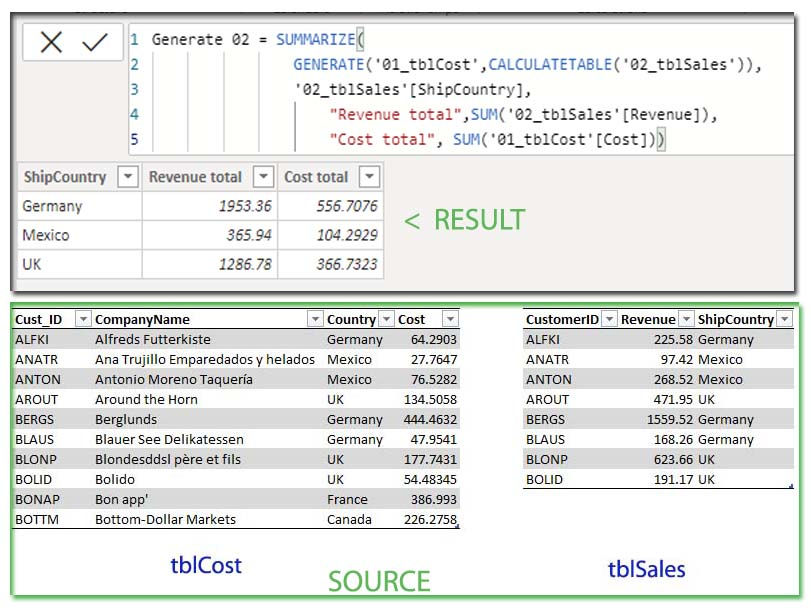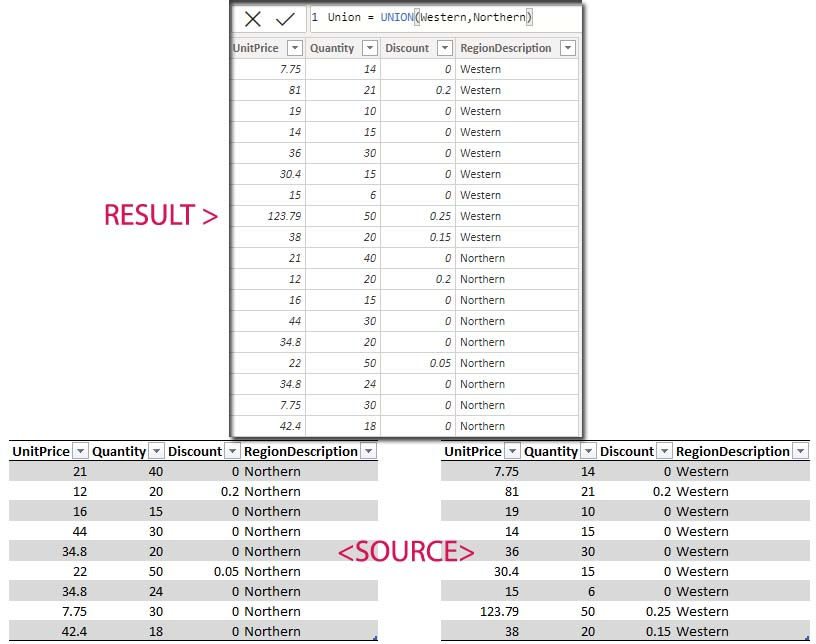top of page
Search
• Marek Vavrovic

# Joins with DAX

Updated: Sep 18, 2021

###### source:## GENERATE, GENERATEALL

Syntax

GENERATE(<table1>, <table2>)

GENERATEALL(<table1>, <table2>)

Return value

A table with the Cartesian product between each row in table1 and the table that results from evaluating table2 in the context of the current row from table1

Notes:

• All column names from table1 and table2 must be different or an error is returned.

• This function is not supported for use in DirectQuery mode when used in calculated columns or row-level security (RLS) rules.

Example 1

Both function return the same result, the cross product of two tables .Example 2

GENARATE with CALCULATETABLE

CalculateTable is a filter function

syntax:

CALCULATETABLE(<expression>[, <filter1> [, <filter2> [, …]]])

I created a left join, means all the rows from tblSales and the matching rows from tblCost. An existing relationship must be between these two tables, otherwise the cartesian product will be returned. GENERATEALL returns the same result.Example 3

If I would switched the table, GENERATEALL returns all the rows including non matching rows, whereas GENERATE returns just the matching rows.Example 4

with SUMMARIZE

Generate =

SUMMARIZE (

GENERATE ( '01_tblCost', CALCULATETABLE ( '02_tblSales' ) ),

'02_tblSales'[ShipCountry],

"Revenue total", SUM ( '02_tblSales'[Revenue] ),

"Cost total", SUM ( '01_tblCost'[Cost] )

)

In this example, first I created an inner join using the GENERATE functionExample 5

with SUMX as a virtual table

Profit =

SUMX (

SUMMARIZE (

GENERATE ( '01_tblCost', CALCULATETABLE ( '02_tblSales' ) ),

'02_tblSales'[ShipCountry],

"Total sales", SUM ( '02_tblSales'[Revenue] ),

"Total expenses", SUM ( '01_tblCost'[Cost] )

),

[Total sales] - [Total expenses]

)## NATURALINNERJOIN vs NATURALLEFTOUTERJOIN

Syntax:

NATURALINNERJOIN(<leftJoinTable>, <rightJoinTable>)

NATURALLEFTOUTERJOIN(<leftJoinTable>, <rightJoinTable>)

Example 1

Inner Join (NATURALINNERJOIN)

There must be a relationship in the data model created, otherwise you will get an error.Example 2

Left Join or Right Join (NATURALLEFTOUTERJOIN)Example 3

NATURALINNERJOIN and NATURALLEFTOUTERJOIN keep all the columns. If you want to retrieve just couple of specific columns, you can use the SELECTCOLUMNS function.Example 4

with FILTER

Left join = FILTER( NATURALLEFTOUTERJOIN('02_tblSales','01_tblCost'), '02_tblSales'[ShipCountry]="Germany")## UNION

SYNTAX:

UNION(<table_expression1>, <table_expression2> [,<table_expression>]…)

• The two tables must have the same number of columns.

• Columns are combined by position in their respective tables.

• The column names in the return table will match the column names in table_expression1.

• Duplicate rows are retained.

Example 1

UNION does the same as Append query in the power query edtor.Example 2

with FILTER## INTERSECT

Returns the row intersection of two tables (of two columns), retaining duplicates.

Syntax:

INTERSECT(<table1>, <table2>)

Return value

A table that contains all the rows in table1 that are also in table2.

Notes

• Intersect(T1, T2) will have a different result set than Intersect(T2, T1).

• Duplicate rows are retained

Example 1

In this example INTERSECT function returned all the rows from table1

and matching rows from table 2Example 2

in this example I have 3 matching rows, the fourth row has a different values in the Number column.Example 3

I want to calculate the customers who purchased some lovely product at least for two calendar weeks in the row. There are 3 of them: John, Steve, Harry. These 3 customers have purchased something every week. Using two tables DimDates containing the continues set of dates and FactSales, a transactional table with the sales for August 2021.Step 1

calculate the total sales.

_TotalSales = SUM(FactSales[Sales])

Step 2

calculate last calendar week sales.

SaleLastWeek =

CALCULATE (

[_TotalSales],

FILTER (

ALL ( DimDates[Week of Year] ),

DimDates[Week of Year]

= MAX ( DimDates[Week of Year] ) - 1))

John, Steve, Harry have a purchase every week in August. Those transactions have been displayed .Step 3

I want to create a measure with two virtual tables. First table will contain all the customers and the second table contains the customers who purchased something in the previous calendar week.Step 4

After I created these two tables, I use them in the measure as a virtual tables to mark the returning customers using some login and INTERSECT function.

Intersect =

VAR AllCustomer = VALUES ( FactSales[Customer] )

VAR CustomerLastWeek =

CALCULATETABLE (

VALUES ( FactSales[Customer] ),

FILTER (

ALL ( DimDates[Week of Year] ),

DimDates[Week of Year]

= MAX ( DimDates[Week of Year] ) - 1

)

)

RETURN

COUNTROWS ( INTERSECT ( CustomerLastWeek, AllCustomer ) )Step 5

creating the total sales of the repeat customers.

Intersect total=

VAR AllCustomer = VALUES ( FactSales[Customer] )

VAR CustomerLastWeek =

CALCULATETABLE (

VALUES ( FactSales[Customer] ),

FILTER (

ALL ( DimDates[Week of Year] ),

DimDates[Week of Year]

= MAX ( DimDates[Week of Year] ) - 1

)

)

RETURN

CALCULATE([_TotalSales]+[SaleLastWeek],

INTERSECT ( CustomerLastWeek, AllCustomer ) )

Steve, John and Harry have an existing transaction every calendar week.